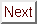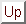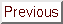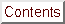Next: Type I Superconductivity Up: Experimental Results: Angular Previous: Experimental Results: Angular

## Extrinsic Influences on Hc2(theta) in Anisotropic Superconductors

As was discussed in Section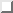, one of the possible extrinsic causes of the deviations in Hc2(theta) is that the GIC's a-axis may not have been mounted perfectly vertically. Figurea) shows the properly aligned case for reference, and Figureb) illustrates the case where the sample is placed inside the inductance bridge with its c-axis rotated by an angle ø from the horizontal. In both a) and b), the laboratory vertical is called ^z. The sample in-plane axes are denoted by ^a and ^a'. Thus in a) ^z and ^a' are parallel, whereas in b), the misaligned case, the angle between ^z and ^a' is also ø. Since the measurements described here were done on HOPG, the sample's c-axis is a well-defined crystallographic direction, but the crystallites are randomly oriented in-plane, and so the directions ^a and ^a' are designated merely for convenience.

The important point to notice here is that in b) the ^a axis is still in the horizontal plane, even though the c-axis is not. Therefore rotation of the sample about ^z will allow correct measurement of Hc2,|| ^a = Hc2,_|_ ^c, but the value measured for Hc2, || ^c will be off be a factor corresponding to ø degrees. In fact, the minimum in the measured Hc2(theta) will come when vecH is in the plane defined by the vector ^c and ^z. Through use of Eqn., one can see that the minimum measured critical field for the misaligned case will be Hc2(0°)/sqrtcos2 ø + epsilon2 sin2 ø. With a bit more effort, by following the original derivation of Morris, Coleman, and Bhandari the formula for Hc2(theta) for a specimen tilted by an angle ø can be derived:The detailed derivation of this formula is outlined in Appendix. If one lets Hc2,|| ^ceff == Hc2,|| ^c/sqrtcos2ø + epsilon2sin2ø and epsiloneff == epsilon/sqrtcos2ø + epsilon2sin2ø, then one recovers the formonly with the effective quantities replacing the actual ones. The impact of a non-zero tilt angle is therefore to increase the measured value of Hc2,|| ^c but not to change the measured value of Hc2,_|_ ^c, therefore reducing the apparent anisotropy, 1/epsilon = Hc2,_|_^c/Hc2,|| ^c. Since the experimental data in Figureb) is higher than the fit near vecH||^c, sample tilt seems like a good candidate for explaining the discrepancy. However, the impact of tilt for a plausible range of ø is quite small since Hc2(theta) is quite flat near theta = 0. The minute amount of distortion of the curves that occurs for a believable tilt angle (ø <= 5°) is demonstrated in Figure. After examining this figure one can conclude that the effect of specimen tilt on Hc2(theta) is probably unimportant.Figure: Why a tilted sample affects the shape of Hc2(theta). The notation ( vecH cdot ^x)^x signifies the projection of vecH along ^x. a) The aligned case. Rotations of the sample around the vertical ^z allow theta to be varied all the way from ( vecH||^c) to ( vecH_|_^c) ( vecH_|_^c = vecH|| ^a). b) The misaligned case. Hc2,_|_^c can still be measured correctly, but instead of the true value of Hc2||^c one will get Hc2,||^c/sqrtcos2ø + epsilon2sin2ø.Figure: The effect of sample tilt on Hc2(theta). The three curves in this picture were calculated using the parameters Hc2,|| ^c = 42 Oe, anisotropy == 1/epsilon = 15 and the following values for the tilt angle: (circ) ø = 0°; (bullet) ø = 10°; (diamond) ø = 40°. The ø = 0° curve corresponds to one of the fits shown in Figureb). Note that the curves for ø = 10° and for ø = 0° are almost indistinguishable.

Besides macroscopic tilt of the specimen, there is another type of misalignment that can influence the shape of the Hc2(theta) curves. A microscopic type of misalignment is mosaic spread, which in this context means the half-width-at-half-maximum-intensity (HWHM) of a GIC's (00l) peaks in a diffractometer rocking scan. The mosaic spread is a measure of the degree to which the c-axes of the crystallites in a piece of HOPG are aligned. Clearly if the planes of a GIC are not flat, the peak in Hc2(theta) will be somewhat smeared out. Therefore, a finite amount of mosaic spread will reduce the anisotropy ratio by reducing Hc2(90°) = Hc2,_|_ ^c. The effect of mosaic spread on the critical fields of the GIC superconductors was estimated by convolving the formula in Equationwith a gaussian function to represent the probability of misalignment as a function of misalignment angle. With a gaussian distribution of misaligned crystallites, for each increment Delta away from perfect alignment, there is a reduction by a factor 1/e in probability. Therefore only orientations within about Delta degrees of perfect alignment contribute significantly. The form of the expression is:where theta is the angle for which the critical field is being calculated, thetai is the dummy variable (which runs from 0° to 180°), and Delta is the mosaic spread in degrees. The results of the calculations are exemplified by the curves in Figure.Figure: The effect of mosaic spread on Hc2(theta), calculated using Equation. The same parameters were used as in Figure, except that here the mosaic spread, Delta, is varied: (circ) Delta = 0°; (bullet) Delta = 3°; (diamond) Delta = 10°.

The most appropriate HWHM of the gaussian would be the mosaic spread measured by (00l) diffraction, if known. In the present work the mosaic spread was unknown, and so Delta was used as a parameter in the fits. Because a non-zero mosaic spread makes the Hc2(theta) curves broader, it actually worsened agreement between experiment and theory, since the theoretical curves are already too broad in the wings of the peak. This finding does not, of course, indicate that the GIC's used in these experiments were perfect crystals; rather it is an indication that the real cause of the unsatisfactory fits for the Tc = 1.5 K samples has not yet been identified.

The presence of multiple crystalline or disordered phases in C4KHg is another property that could impact upon the angular dependence curves. The differing properties of the two phases are discussed in detail in Chapter. As far as the Hc2(theta) curves go, it suffices to say that since the phases may have different Tc's, they could have different upper critical fields. If these two phases are distributed inhomogenenously in the GIC's, then at the lowest temperatures, where both of them are superconducting, their presence could distort Hc2(theta). However, the temperature and sample dependence of the quality of the fits weigh against this interpretation. For one thing, Figureb) shows that the Hc2(theta) curves are quite reproducible from sample to sample, an unlikely occurrence if the distribution of phases is important. Secondly, as mentioned in connection with Figure, the quality of the fits improves with decreasing temperature, the opposite of what would be expected if a lower- Tc minority phase were causing the deviations. Thirdly, the samples which show larger deviations from the AGL functional form have narrower zero-field transitions than the lower- Tc specimens which are well-fit by Eqn.. X-ray and neutron diffraction experiments confirm that the higher- Tc samples are actually more uniform than the lower- Tc ones.

The last of the extrinsic factors that needs to be considered as a explanation of the disagreement between the Hc2(theta) data and fits to the data is the possibility of bias influenced by the method of data reduction. As was discussed in Section, the standard working definition of Hc2 (for inductive transitions) was used; this procedure gives Hc2 as the intersection of a tangent drawn to the most linear part of the field sweep with the level upper portion of the trace. The unavoidable question when encountering difficulties in fitting Hc2 data is whether there is any reason to expect agreement given that the theoretical definition of Hc2 is so different from the working one. That is, there is no guarantee that the field found by the procedure described above corresponds to the highest field where vortices can nucleate in the superconductor, which is the theoretical definition of Hc2.[252,108] The possibility of bias due to analysis is particularly troublesome for the study of Hc2 in anisotropic superconductors, where the transition shape can be a strong function of theta, as Figureb) shows.

The only response that an experimentalist can make to such criticism is that the procedure defined above is as good as any available, and that it is capable of producing data which are well-fit by Equation, as Figurea) shows. In order to check for any bias introduced by the tangent method of critical field determination used here, the alternative definition of Hc2 as the 90% completion point of the transition was also tried in analyzing some Hc2(theta) data sets. Figureis a comparison of the curves obtained using the two different analysis techniques. As this juxtaposition shows, slightly different curves are produced by the two analysis methods. However, the agreement with Eqn.is not improved. Therefore it is thought that the unsatisfactory quality of the fits to the Tc = 1.5 K samples is not an artifact of the analysis. In a similar vein, Decroux and Fischer have noted that Hc2 results on the molybdenum chalcogenide compounds (also called Chevrel phases) are not biased by the critical field definition as long as the compounds do not contain magnetic ions.Figure: Comparison of the effect of the two definitions of Hc2 on Hc2(theta). circ, tangent definition; diamond, 90% definition. Data are for a Tc = 1.53 K sample at T/T c = 0.29. The 90% method tends to produce slightly higher critical fields, but there is only a small difference between the shapes of the curves for the two analysis methods. Use of the 90% definition does not improve agreement with Eqn..Next: Type I Superconductivity Up: Experimental Results: Angular Previous: Experimental Results: Angular

alchaiken@gmail.com (Alison Chaiken)
Wed Oct 11 22:59:57 PDT 1995